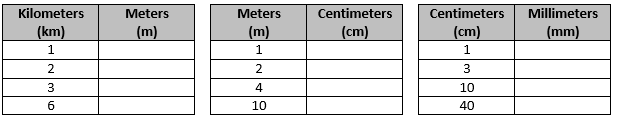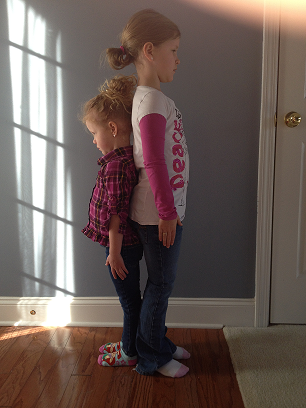# Unit Conversions

## Objective

Express metric length measurements in terms of a smaller unit, recording measurement equivalents in a two-column table. Solve one-step word problems that require metric length unit conversion.

## Materials and Resources

• Meterstick  — (Optional: 1 per teacher/student or group of students)

## Common Core Standards

### Core Standards

?

• 4.MD.A.1 — Know relative sizes of measurement units within one system of units including km, m, cm; kg, g; lb, oz.; l, ml; hr, min, sec. Within a single system of measurement, express measurements in a larger unit in terms of a smaller unit. Record measurement equivalents in a two column table. For example, know that 1 ft is 12 times as long as 1 in. Express the length of a 4 ft snake as 48 in. Generate a conversion table for feet and inches listing the number pairs (1, 12), (2, 24), (3, 36), …

• 4.MD.A.2 — Use the four operations to solve word problems involving distances, intervals of time, liquid volumes, masses of objects, and money, including problems involving simple fractions or decimals, and problems that require expressing measurements given in a larger unit in terms of a smaller unit. Represent measurement quantities using diagrams such as number line diagrams that feature a measurement scale.

?

• 3.MD.A.2

• 4.OA.A.2

## Criteria for Success

?

1. Establish benchmarks for the metric units of a kilometer, meter, centimeter, and millimeter.
2. Understand that a kilometer is 1,000 times longer than a meter, a meter is 100 times longer than a centimeter, and a centimeter is 10 times longer than a millimeter.
3. Use these relationships to deduce conversion rates in the metric system of measurement not otherwise mentioned, e.g., there are 100,000 centimeters in a kilometer (MP.7, MP.8).
4. Use these relationships to convert measurements from a larger metric length unit to a smaller unit (MP.7, MP.8).
5. Use these relationships to convert measurements from mixed metric length units to a smaller unit (MP.7, MP.8).
6. Solve one-step word problems that require metric length unit conversions (MP.4).

## Tips for Teachers

?

• Before starting the whole unit, it may be helpful to get a sense of students’ benchmarks for various measurement units. You can use select tasks from this NRICH task to do so: Discuss and Choose.
• Note that in this lesson and the following one, only the units mentioned in the Progressions for the Common Core State Standards in Mathematics, K-5 Geometric Measurement document are included, such as km, m, cm, and mm, but not other units such as hectometers, decameters, or decimeters. You may decide to modify Lessons 1 and 2 to include those units. But, since they are not explicitly mentioned as part of the Grade 4 expectations and are used in much lower frequency in everyday life compared to the ones included in this lesson, they have been excluded.
• The work that students do with measurement conversion (4.MD.1) in Lessons 1, 2, 5, 6, and 7 is connected to the idea of “times as much” in multiplication (4.OA.1). Thus, many tasks and guiding questions below draw attention to this language specifically to make that connection more apparent.

#### Fishtank Plus

• Problem Set
• Student Handout Editor
• Vocabulary Package

?

### Problem 1

Find the millimeter, centimeter, and meter measurement on the meter stick.

a. What do you notice about the relationship between the length of a millimeter and the length of a centimeter?

b. What do you notice about the relationship between the length of a centimeter and the length of a meter?

c. What do you notice about the relationship between the length of a millimeter and the length of a meter?

### Problem 2

Fill in the following conversion tables.### Problem 3

Mr. Duffy wants to buy a new pair of skis. Skis are sold based on their length in centimeters. If Mr. Duffy wants to buy a pair of skis that are his exact height, and he is 1 meter 83 centimeters tall, what length skis should Mr. Duffy buy?

### Problem 4

Two sisters stand back to back to see how much taller the older sister is than the younger sister.The older sister is 1 m 30 cm tall. The younger sister is 24 cm 2 mm shorter than her. How tall is the younger sister?

#### References

Questioning My Metacognition Lil' Sister

Lil' Sister by Graham Fletcher is made available on Questioning My Metacognition under the CC BY-SA 4.0 license. Accessed June 13, 2018, 12:52 p.m..

Modified by The Match Foundation, Inc.

## Discussion of Problem Set

?

• What pattern did you notice in the equivalences for #2 and #4? How did converting 1 kilometer to 1,000 meters in #1(a) help you to solve #2(a)?
• How long is the ribbon in #3 in centimeters? In millimeters?
• How does the ruler in #8 show that a centimeter is ten times as large as a millimeter?
• What is wrong with Jason’s reasoning in #10? How much larger is a kilometer than a millimeter? How do you know?

?

### Problem 1

Complete the conversion table.

Distance

 71 km _____ m 30 km _____ m 81 m _____ cm 4 m _____ cm 50 cm _____ mm 13 m _____ mm

#### References

EngageNY Mathematics Grade 4 Mathematics > Module 2 > Topic A > Lesson 1Exit Ticket, Question #1

Grade 4 Mathematics > Module 2 > Topic A > Lesson 1 of the New York State Common Core Mathematics Curriculum from EngageNY and Great Minds. © 2015 Great Minds. Licensed by EngageNY of the New York State Education Department under the CC BY-NC-SA 3.0 US license. Accessed Dec. 2, 2016, 5:15 p.m..

Modified by The Match Foundation, Inc.

### Problem 2

13 km 20 m = ___________ m

#### References

EngageNY Mathematics Grade 4 Mathematics > Module 2 > Topic A > Lesson 1Exit Ticket, Question #2

Grade 4 Mathematics > Module 2 > Topic A > Lesson 1 of the New York State Common Core Mathematics Curriculum from EngageNY and Great Minds. © 2015 Great Minds. Licensed by EngageNY of the New York State Education Department under the CC BY-NC-SA 3.0 US license. Accessed Dec. 2, 2016, 5:15 p.m..

### Problem 3

The female world record for high jump is 2 meters and 9 centimeters. How high is that in centimeters?

?